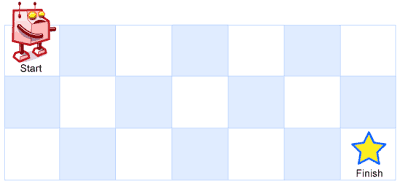# Question

Formatted question description: https://leetcode.ca/all/62.html

There is a robot on an m x n grid. The robot is initially located at the top-left corner (i.e., grid). The robot tries to move to the bottom-right corner (i.e., grid[m - 1][n - 1]). The robot can only move either down or right at any point in time.

Given the two integers m and n, return the number of possible unique paths that the robot can take to reach the bottom-right corner.

The test cases are generated so that the answer will be less than or equal to 2 * 109.

Example 1:Input: m = 3, n = 7
Output: 28


Example 2:

Input: m = 3, n = 2
Output: 3
Explanation: From the top-left corner, there are a total of 3 ways to reach the bottom-right corner:
1. Right -> Down -> Down
2. Down -> Down -> Right
3. Down -> Right -> Down


Constraints:

• 1 <= m, n <= 100

# Algorithm

Using Dynamic Programming to solve, you can maintain a two-dimensional array dp, where dp[i][j] represents the number of different moves to the current position, and then the state transition equation can be obtained as: dp[i][j ] = dp[i-1][j] + dp[i][j-1], here in order to save space, use a one-dimensional array dp, refresh line by line.

# Code

• 
public class Unique_Paths {

class Solution {
public int uniquePaths(int m, int n) {

if (m <= 0 || n <= 0) {
return 0;
}

int[][] dp = new int[m][n];

for (int i = 0; i < m; i++) {
for (int j = 0; j < n; j++) {

if (i == 0 || j == 0) {
dp[i][j] = 1;
} else { // i,j both not 1
dp[i][j] = dp[i-1][j] + dp[i][j-1];
}
}
}

return dp[m-1][n-1];
}
}

}

############

class Solution {
public int uniquePaths(int m, int n) {
int[][] dp = new int[m][n];
for (int i = 0; i < m; ++i) {
Arrays.fill(dp[i], 1);
}
for (int i = 1; i < m; ++i) {
for (int j = 1; j < n; ++j) {
dp[i][j] = dp[i - 1][j] + dp[i][j - 1];
}
}
return dp[m - 1][n - 1];
}
}

• // OJ: https://leetcode.com/problems/unique-paths/
// Time: O(MN)
// Space: O(N)
class Solution {
public:
int uniquePaths(int m, int n) {
vector<int> dp(n + 1, 0);
dp[n - 1] = 1;
for (int i = m - 1; i >= 0; --i) {
for (int j = n - 1; j >= 0; --j) dp[j] += dp[j + 1];
}
return dp;
}
};

• class Solution:
def uniquePaths(self, m: int, n: int) -> int:
# avoid setting dp[][] to 1 for i==0 or j==0 as initialization
dp = [ * n for _ in range(m)]
for i in range(1, m):
for j in range(1, n):
dp[i][j] = dp[i - 1][j] + dp[i][j - 1]
return dp[-1][-1]

############

class Solution(object):
def uniquePaths(self, m, n):
"""
:type m: int
:type n: int
:rtype: int
"""
dp =  * n

for i in range(1, m):
pre = 1
for j in range(1, n):
dp[j] = dp[j] + pre
pre = dp[j]
return dp[-1]


• func uniquePaths(m int, n int) int {
dp := make([][]int, m)
for i := 0; i < m; i++ {
dp[i] = make([]int, n)
}
for i := 0; i < m; i++ {
for j := 0; j < n; j++ {
if i == 0 || j == 0 {
dp[i][j] = 1
} else {
dp[i][j] = dp[i-1][j] + dp[i][j-1]
}
}
}
return dp[m-1][n-1]
}

• function uniquePaths(m: number, n: number): number {
let dp = Array.from({ length: m }, v => new Array(n).fill(1));
for (let i = 1; i < m; ++i) {
for (let j = 1; j < n; ++j) {
dp[i][j] = dp[i - 1][j] + dp[i][j - 1];
}
}
return dp[m - 1][n - 1];
}


• impl Solution {
pub fn unique_paths(m: i32, n: i32) -> i32 {
let (m, n) = (m as usize, n as usize);
let mut dp = vec![1; n];
for i in 1..m {
for j in 1..n {
dp[j] += dp[j - 1];
}
}
dp[n - 1]
}
}


• /**
* @param {number} m
* @param {number} n
* @return {number}
*/
var uniquePaths = function (m, n) {
const f = Array(m)
.fill(0)
.map(() => Array(n).fill(1));
for (let i = 1; i < m; ++i) {
for (let j = 1; j < n; ++j) {
f[i][j] = f[i - 1][j] + f[i][j - 1];
}
}
return f[m - 1][n - 1];
};